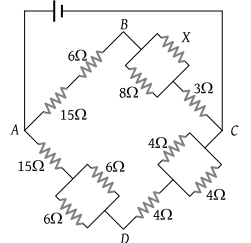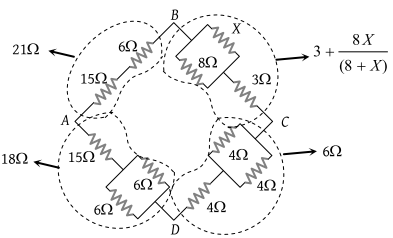# NEET Questions Solved

In the figure given the value of X resistance will be, when the p.d. between B and D is zero(1) 4 ohm

(2) 6 ohm

(3) 8 ohm

(4) 9 ohm

(3) Potential difference between B and D is zero, it means Wheatstone bridge is in balanced conditionSo $\frac{P}{Q}=\frac{R}{S}$

$\frac{21}{3+\frac{8X}{\left(8+X\right)}}=\frac{18}{6}$

$X=8\Omega$

Difficulty Level:

• 10%
• 20%
• 66%
• 6%